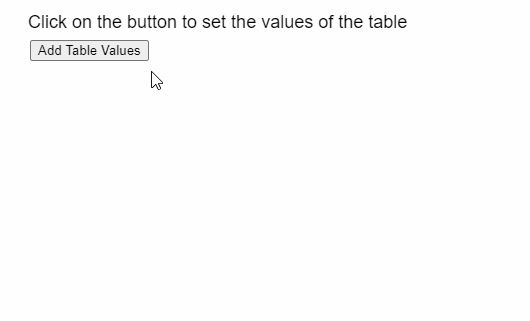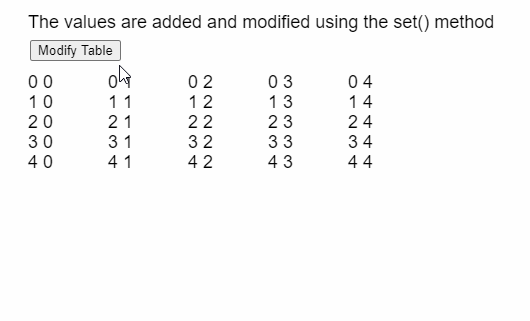# p5.Table set() Method

• Last Updated : 07 Jul, 2020

The set() method of p5.Table in p5.js is used to store the given value to the given row and column of the table. The row can be specified by the row ID and the column can be specified by its column ID or name.

Syntax:

Hey geek! The constant emerging technologies in the world of web development always keeps the excitement for this subject through the roof. But before you tackle the big projects, we suggest you start by learning the basics. Kickstart your web development journey by learning JS concepts with our JavaScript Course. Now at it's lowest price ever!

`set( row, column, value )`

Parameters: This function accepts three parameters as mentioned above and described below:

• row: It is a Number that denotes the row ID of the row.
• column: It is a String or Number that denotes the column name or ID of the column.
• value: It is a String or Number that specifies the value that has to be stored.

Below example illustrates the set() method in p5.js:

Example 1:

 `function` `setup() {``  ``createCanvas(500, 300);``  ``textSize(18);`` ` `  ``text(``"Click on the button to set the"` `+``       ``" values of the table"``, 20, 20);`` ` `  ``setBtn = createButton(``"Add Table Values"``);``  ``setBtn.position(30, 40);``  ``setBtn.mouseClicked(setTableData);`` ` `  ``// Create the table``  ``table = ``new` `p5.Table();`` ` `  ``// Add 5 columns and rows to the table``  ``for` `(let i = 0; i < 5; i++) {``    ``table.addColumn(``"Column "` `+ i);``    ``table.addRow();``  ``}``}`` ` `function` `setTableData() {``  ``for` `(let r = 0; r < 5; r++) {``    ``for` `(let c = 0; c < 5; c++) {`` ` `      ``// Set the value at the given``      ``// column and row of the table``      ``table.set(r, c, r + ``" "` `+ c);``    ``}``  ``}`` ` `  ``showTable();``}`` ` `function` `showTable() {``  ``clear();``    ``text(``"Click on the button to set the"` `+``       ``" values of the table"``, 20, 20);`` ` `  ``// Show all the columns present``  ``for` `(let c = 0; c < 5; c++) {``    ``text(table.columns, 20 + 80 * c, 80);``  ``}`` ` `  ``// Show all the rows currently``  ``// present in the table``  ``for` `(let r = 0; r < 5; r++) {``    ``for` `(let c = 0; c < 5; c++) {``      ``text(table.getString(r, c),``           ``20 + 80 * c,``           ``100 + 20 * r);``    ``}``  ``}``}`

Output:Example 2:

 `function` `setup() {``  ``createCanvas(500, 300);``  ``textSize(18);`` ` `  ``changeBtn =``    ``createButton(``"Modify Table"``);``  ``changeBtn.position(30, 40);``  ``changeBtn.mouseClicked(modifyTable);`` ` `  ``// Create the table``  ``table = ``new` `p5.Table();`` ` `  ``// Add 5 columns and rows to the table``  ``for` `(let i = 0; i < 5; i++) {``    ``table.addColumn(``"Column "` `+ i);``    ``table.addRow();``  ``}`` ` `  ``setTableData();`` ` `  ``// Display the table``  ``showTable();``}`` ` `function` `setTableData() {``  ``for` `(let r = 0; r < 5; r++) {``    ``for` `(let c = 0; c < 5; c++) {`` ` `      ``// Set the value at the given``      ``// column and row of the table``      ``table.set(r, c, r + ``" "` `+ c);``    ``}``  ``}`` ` `  ``showTable();``}`` ` `function` `modifyTable() {``  ``for` `(let r = 0; r < 5; r++) {``    ``for` `(let c = 0; c < 5; c++) {`` ` `      ``// If the index of the table is``      ``// a diagonal, set it to "XX"``      ``if` `(r == c) table.set(r, c, ``"XX"``);``    ``}``  ``}`` ` `  ``showTable();``}`` ` `function` `showTable() {``  ``clear();``   ``text(``"Click on the button to set the"` `+``       ``" values of the table"``, 20, 20);`` ` `  ``// Show all the rows currently``  ``// present in the table``  ``for` `(let r = 0; r < 5; r++) {``    ``for` `(let c = 0; c < 5; c++) {``      ``text(table.getString(r, c),``           ``20 + 80 * c,``           ``80 + 20 * r);``    ``}``  ``}``}`

Output:Online editor: https://editor.p5js.org/

My Personal Notes arrow_drop_up